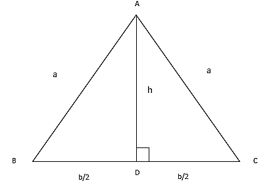# Area of Isosceles Triangle Formula

## Area of Isosceles Triangle Formula with Solved Examples

Measurements related to Isosceles Triangles
An isosceles triangle is one in which two sides are equal in length. By this definition , an equilateral triangle is also an isosceles triangle. Let us consider an isosceles triangle as shown in the following diagram (whose sides are known, say a, a and b).As the altitude of an isosceles triangle drawn from its vertical angle is also its angle bisector and the median to the base (which can be proved using congruence of triangles), we have two right triangles as shown in the figure above.
Using the Pythagorean theorem, we have the following result.
$A{C^2} = A{D^2} + D{C^2} \Rightarrow {h^2} = {a^2} - {\left( {\frac{b}{2}} \right)^2} \Rightarrow h = \sqrt {{a^2} - \frac{{{b^2}}}{4}}$
So the area of the isosceles can be calculated as follows.
${\text{area}} = \frac{1}{2}bh = \frac{b}{2}\sqrt {{a^2} - \frac{{{b^2}}}{4}}$
The perimeter of the isosceles triangle is relatively simple to calculate, as shown below.
${\text{perimeter}} = 2a + b$
Also note that the area of the isosceles triangle can be calculated using Heron’s formula.
$s = \frac{{a + a + b}}{2} = \frac{{2a + b}}{2} = a + \frac{b}{2}$
$\begin{gathered} {\text{area}} = \sqrt {\left( {a + \frac{b}{2}} \right)\left( {a + \frac{b}{2} - a} \right)\left( {a + \frac{b}{2} - b} \right)} \hfill \\ \,\,\,\,\,\,\,\,\,\,\, = \sqrt {\left( {a + \frac{b}{2}} \right)\left( {\frac{b}{2}} \right)\left( {a - \frac{b}{2}} \right)} = \frac{b}{2}\sqrt {{a^2} - \frac{{{b^2}}}{4}} \hfill \\ \end{gathered}$
Trigonometry can also be used in the case of isosceles triangles more easily because of the congruent right triangles.
Let’s look at an example to see how to use these formulas.

Question: If the base and the area of an isosceles triangle are respectively $8\,cm$ and $12\,c{m^2}$, then find its perimeter.
Solution:
$b = 4\,cm,\,\,area = 12\,c{m^2}$
$\begin{gathered} area = \frac{b}{2}\sqrt {{a^2} - \frac{{{b^2}}}{4}} \hfill \\ \Rightarrow 12 = \frac{8}{2}\sqrt {{a^2} - \frac{{{8^2}}}{4}} \hfill \\ \Rightarrow 3 = \sqrt {{a^2} - 16} \Rightarrow {a^2} = 25 \Rightarrow a = 5\,cm \hfill \\ \end{gathered}$
Thus the perimeter of the isosceles triangle is calculated as follows.
$perimeter = 2a + b = 2 \times 5 + 8 = 18\,cm$
Why don’t you try solving the following sum to see if you have mastered using these formulas?
Question: Calculate the area of an isosceles triangle whose sides are 13 cm, 13 cm and 24 cm.
Options:
(a) $60\,c{m^2}$
(b) $45\,c{m^2}$
(c) $30\,c{m^2}$
(d) none of these
$a = 13\,cm,\,\,b = 24\,cm$
$\begin{gathered} area = \frac{b}{2}\sqrt {{a^2} - \frac{{{b^2}}}{4}} \hfill \\ \,\,\,\,\,\,\,\,\,\, = \frac{{24}}{2}\sqrt {{{13}^2} - \frac{{{{24}^2}}}{4}} = 12 \times \sqrt {169 - 144} = 12 \times 5 = 60\,c{m^2} \hfill \\ \end{gathered}$